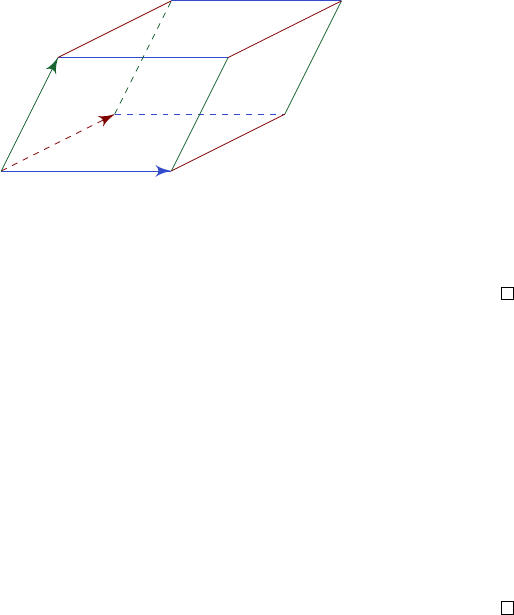2Vectors

IA Vectors and Matrices2.5 Scalar triple product
Definition (Scalar triple product). The scalar triple product is defined as
[a, b, c] = a · (b × c).
Proposition.
If a parallelepiped has sides represented by vectors
a, b, c
that
form a right-handed system, then the volume of the parallelepiped is given by
[a, b, c].
b
c
a
Proof.
The area of the base of the parallelepiped is given by
|b||c|sin θ
=
|b × c|
.
Thus the volume=
|b × c||a|cos φ
=
|a · (b × c)|
, where
φ
is the angle between
a
and the normal to
b
and
c
. However, since
a, b, c
form a right-handed system,
we have a · (b × c) 0. Therefore the volume is a · (b × c).
Since the order of a, b, c doesn’t affect the volume, we know that
[a, b, c] = [b, c, a] = [c, a, b] = [b, a, c] = [a, c, b] = [c, b, a].
Theorem. a × (b + c) = a × b + a × c.
Proof. Let d = a ×(b + c) a ×b a ×c. We have
d · d = d · [a × (b + c)] d · (a × b) d · (a × c)
= (b + c) · (d × a) b · (d × a) c · (d × a)
= 0
Thus d = 0.